# 1.统计每个班同学各科成绩平均分大于80分的人数和人数占比

• 收获
有筛选条件的统计数量问题的万能模板
select sum(
case when <判断表达式> then 1
else 0
end
) as 数量
from 信息表；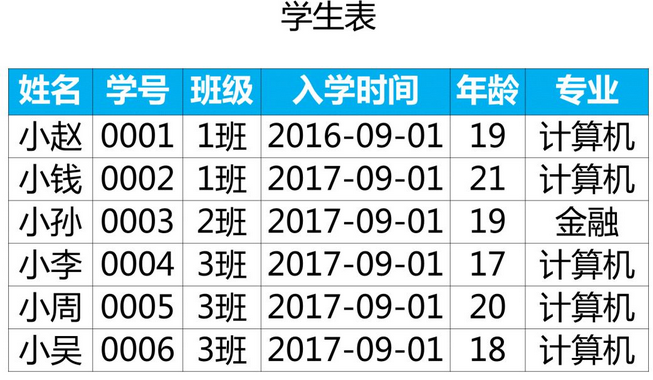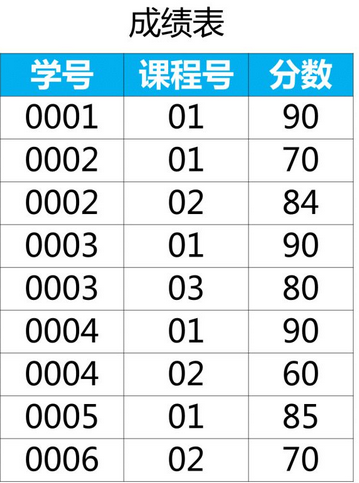##### 思路：

1.每个同学的平均分【临时表】

select 学号,
avg(分数) 平均成绩
from 成绩表
group by 学号;


2.各科平均成绩大于80分的人数

select sum(
case when a.平均成绩>80 then 1 else 0 end) 人数
from
(select 学号,
avg(分数) 平均成绩
from 成绩表
group by 学号) a;


3.各科平均成绩大于80分的人数的占比

select sum(
case when a.平均成绩>80 then 1 else 0 end )/count(a.学号) 占比
from
(select 学号,
avg(分数) 平均成绩
from 成绩表
group by 学号) a;


4.学生表左连接临时表，按班级分组

select a.班级,
sum(case when b.平均成绩>80 then 1 else 0 end) 人数,
sum(case when b.平均成绩>80 then 1 else 0 end)/count(a.学号) 人数占比
from 学生表 a
left join
(select 学号,avg(分数) 平均成绩
from 成绩表
group by 学号 ) b
on a.学号=b.学号
group by a.班级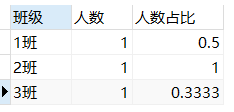# 2.某网站有顾客表和消费表，请统计每个城市的顾客平均消费在1000元以上的人数，输出城市，人数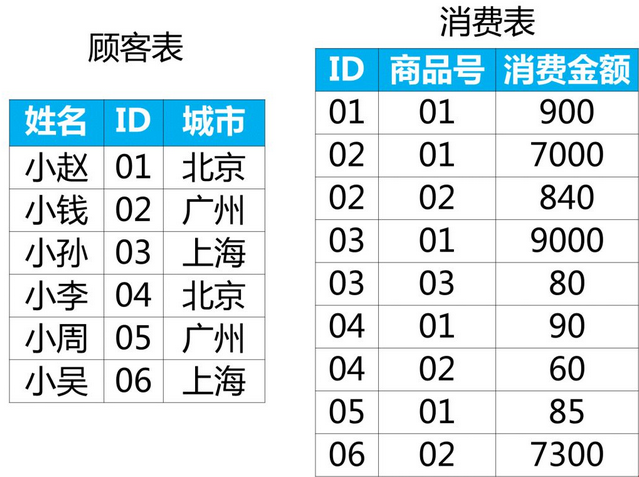select a.城市,
sum(case when b.平均消费>1000 then 1 else 0 end) 人数
from 顾客表 a
left join
(select ID,avg(消费金额) as 平均消费
from 消费表
group by ID) b
on a.ID=b.ID
group by 城市;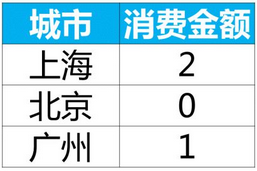# 3.行列互换

select 学号,
max(case 课程 when '语文' then 成绩,else 0 end) 语文成绩,
max(case 课程 when '数学' then 成绩,else 0 end) 数学成绩
from 成绩表
group by 学号;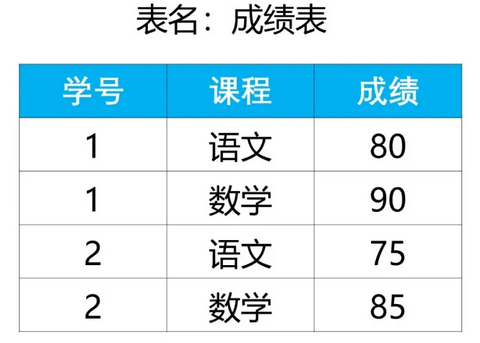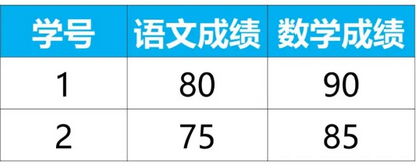##### 模板：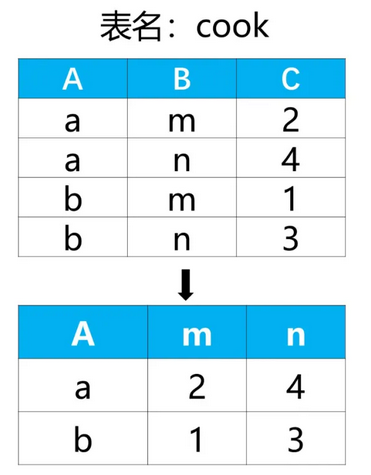select A,
-- 第2步，在行列互换结果表中，其他列里的值分别使用case和max来获取
max(case B when 'm' then C else 0 end) as 'm',
max(case B when 'n' then C else 0 end) as 'n'
from cook
-- 第1步，在行列互换结果表中按第1列分组
group by A;


# 4.复杂业务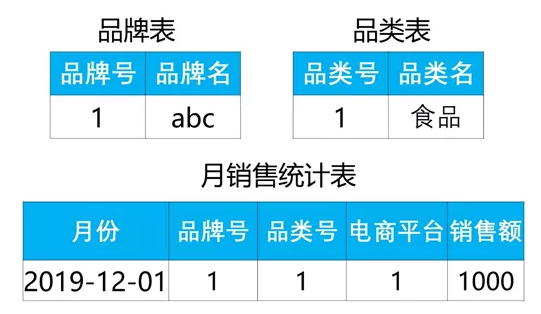• 1.对于指定品类号范围（品类号列表：12，33，45，99，1001），查询2019年每个电商平台上每个品牌号对应每个品类号的累计销售额
品牌号 品牌名 品类号 品类名 电商平台 销售额
select a.品牌号,b.品牌名,
a.品类号,c.品类名,
a.电商平台,a.销售额
from
(select 电商平台,品牌号,品类号,sum(销售额) 销售额
from 月销售统计表
where 品类号 in (12,33,45,99,1001)
and year(月份)='2019'
group by 电商平台,品牌号,品类号) a
left join 品牌表 b
on a.品牌号=b.品牌号
left join 品类表 c
on a.品类号=c.品类号;

• 2.查询2019年有5个以上(含5个)不同 品类号 的 单月单平台销售额大于等于10000的品牌列表，及对应的不同品类号 数量
品牌号 品牌名 品类数量

### 不要忘记 distinct 品类号

'''

1.单品牌单月单平台销售额>=10000
2.单品牌有>=5个不同的品类数

select 品牌号,
count(distinct 品类号) 品类数量
from 月销售统计表
where year(月份)='2019'
group by 品牌号,月份,电商平台
having sum(销售额)>=10000
and count(distinct 品类号)>=5;
'''

select a.品牌号,
b.品牌名,
a.品类号数量
from
(select 品牌号,
count(distinct 品类号) 品类号数量
from 月销售统计表
where year(月份)='2019'
group by 品牌号,月份,电商平台
having sum(销售额)>=10000
and count(distinct 品类号)>=5) a
left join 品牌表 b
on a.品牌号=b.品牌号;

• 3.查询2019年只在电商平台1上有销售额的品牌中（即排除电商平台为2时销售额累计大于0的品牌），电商平台1的累计销售额最大的Top30个品牌及对应的销售额
品牌号 品牌名 平台1总销售额
1.找1平台销售额大于0且2平台销售额等于0的品牌
select a.品牌号
from
(select 品牌号
from 月销售统计表
where year(月份)='2019' and 电商平台='1'
group by 品牌号
having sum(销售额)>0) a
join
(select 品牌号
from 月销售统计表
where year(月份)='2019' and 电商平台='2'
group by 品牌号
having sum(销售额)=0) b
on a.品牌号=b.品牌号

select a.品牌号,
c.品牌名,
a.销售额 销售总额
from
(select 品牌号,
sum(销售额) 销售额
from 月销售统计表
where year(月份)='2019' and 电商平台='1'
group by 品牌号
having sum(销售额)>0) a
join
(select 品牌号
from 月销售统计表
where year(月份)='2019' and 电商平台='2'
group by 品牌号
having sum(销售额)=0) b
on a.品牌号=b.品牌号
join 品牌表 c
on a.品牌号=c.品牌号
order by a.销售额 desc
limit 30

• 4.查询2019年在两个电商平台中分别同时都能进入销售额Top 50的品牌及对应的全电商平台累计销售额
品牌号 品牌名 全平台累计销售额
1.品牌号
select a.品牌号
from
(select 品牌号
from 月销售统计表
where year(月份)='2019' and 电商平台='1'
group by 品牌号
order by sum(销售额) desc
limit 50) a
join
(select 品牌号
from 月销售统计表
where year(月份)='2019' and 电商平台='2'
group by 品牌号
order by sum(销售额) desc
limit 50) b
on a.品牌号=b.品牌号

select a.品牌号,
c.品牌名,

from
(select 品牌号,
sum(销售额) 电商平台1
from 月销售统计表
where year(月份)='2019' and 电商平台='1'
group by 品牌号
order by sum(销售额) desc
limit 50) a
join
(select 品牌号,
sum(销售额) 电商平台2
from 月销售统计表
where year(月份)='2019' and 电商平台='2'
group by 品牌号
order by sum(销售额) desc
limit 50) b
on a.品牌号=b.品牌号
join 品牌表 c
on a.品牌号=c.品牌号;


# 5.用户访问次数表，列名包括用户编号、用户类型、访问量。要求在剔除访问次数前20%的用户后，每类用户的平均访问次数。（拼多多、网易面试题）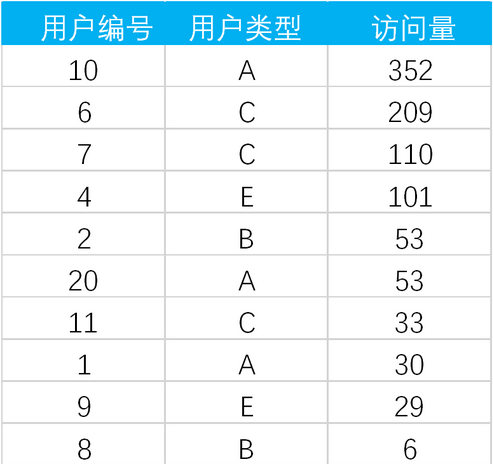select 用户类型,
avg(访问量) 平均访问次数
from
(select *,
ntile(5) over(order by 访问量 desc) rank
from 用户访问次数表) a
where a.rank !=1
group by 用户类型;


# 6.查询每门课程的前3高成绩。注意：如果出现并列第一的情况，则同为第一名。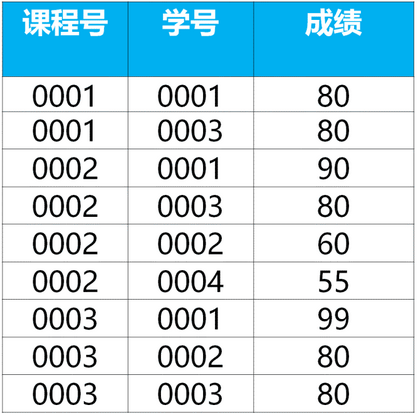select 课程号,

from
(select *,
dese_rank() over(partition by 课程号 order by 成绩 desc) 排名
from 成绩表) a
where a.排名<=3;

'''

select *
from
(select *,
row_number() over (partition by 要分组的列名
order by 要排序的列名 desc) 排名
from 表名) a
where 排名 <= N;

'''


# 7.查找出每门课程中成绩最好的学生的姓名和该学生的课程及成绩。需要注意：可能出现并列第一的情况。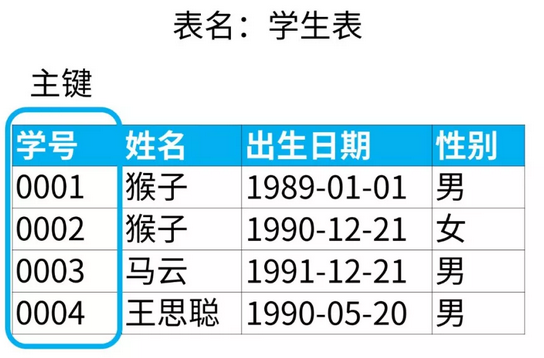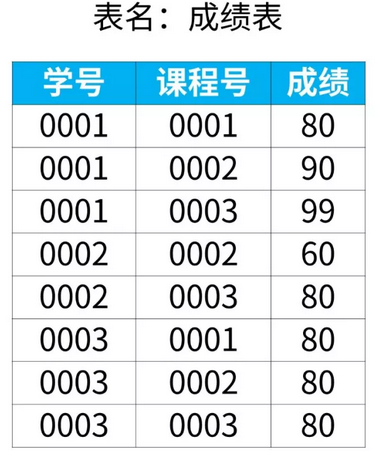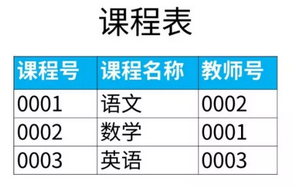当两列同时作为关键字段进行条件查询时
select c.学号,c.姓名,b.课程,a.成绩
from 成绩表 a
left join 课程表 b on a.课程号=b.课程号
left join 学生表 c on a.学号=c.学号
where (a.课程号,a.成绩) in
(select 课程号,max(成绩) from 成绩表 group by 课程号);


# 8.查找当前所有雇员入职以来的薪水涨幅，给出雇员编号以及其对应的薪水涨幅，并按照薪水涨幅进行升序。（注：薪水表中结束日期为2004-01-01的才是当前员工，否则是已离职员工）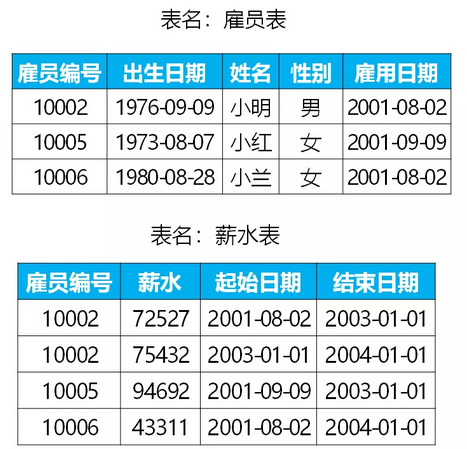薪水涨幅=当前薪水-入职薪水
1.当前薪水，日期为'2004-01-01'的员工的薪水
select 雇员编号,薪水 当前薪水
from 薪水表
where 结束日期='2004-01-01'
2.入职薪水，雇员表中雇用日期=新水表中起始日期
select a.雇员编号,
b.薪水 入职薪水
from 雇员表 a
left join 薪水表 b
on a.雇佣日期=b.起始日期

select m.雇员编号,

from
(select 雇员编号,薪水 当前薪水
from 薪水表
where 结束日期='2004-01-01') m
left join
(select a.雇员编号,
b.薪水 入职薪水
from 雇员表 a
left join 薪水表 b
on a.雇员编号=b.雇员编号
where a.雇佣日期=b.起始日期
and a.雇员编号 in
(select 雇员编号
from 薪水表
where 结束日期 = '2004-01-01')) n
on m.雇员编号=n.雇员编号


# 9.找出语文课中成绩第二高的学生成绩。如果不存在第二高成绩的学生，那么查询应返回 null。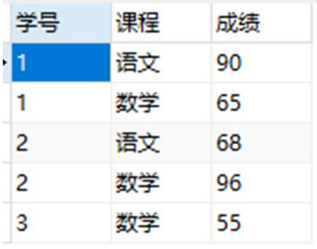'''

===========================================
id:1-100
limit 10,1【11】
limit 10,3【11,12,13】
limit 10 offset 2 【3,4,5,6,7,8,9,10,11,12】

===========================================
'''

2.1.找出语文课成绩最高的学生成绩
2.2.查找语文课成绩第二名

select max(distinct 成绩)
from 成绩表
where 课程='语文' and
'成绩'<
(select max(distinct 成绩)
from 成绩表
where 课程='语文')

select distinct 成绩
from 成绩表
where 课程='语文'
order by 课程,成绩 desc
limit 1,1

ifnull(a,b)

select
ifnull((select distinct 成绩
from 成绩表
where 课程='语文'
order by 课程,成绩 desc
limit 1,1),null) 语文课第二名成绩;

select
ifnull
((select distinct Salary
from Employee
order by Salary desc
limit 1,1),null)
SecondHighestSalary；


# 10.建表相关

建表语句:
CREATE TABLE 学生表 (
学号 int(11) NOT NULL AUTO_INCREMENT,
姓名 varchar(50) DEFAULT NULL,
班级 varchar(5) DEFAULT NULL,
PRIMARY KEY (学号)
) ENGINE=InnoDB AUTO_INCREMENT=2 DEFAULT CHARSET=utf8

INSERT INTO 学生表 (学号,姓名,班级)
VALUES(0003,'小孙','2班');
INSERT INTO 学生表 (学号,姓名,班级)

DELETE FROM table_name

drop table table_name

10-0610-19
03-052145
03-05173
08-221918
05-1267
07-12299
02-07235